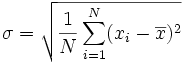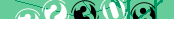# PHP: Useful Functions

A collection of miscellaneous functions that you may find useful.

## Calculating standard deviation

This function returns the standard deviation of an array of values, based on the equation:(image source: Wikipedia)

``` function standard_deviation(\$arr) { \$N = count(\$arr); \$mean = array_sum(\$arr) / \$N; \$sum = 0; foreach(\$arr as \$val) \$sum += pow(\$val - \$mean, 2); return sqrt(\$sum / \$N); }```

## Calculating distances

This function takes as inputs the latitude and logitude coordinates of two points (decimal format) and returns an approximation of the (great circle) distance between those points in kilometers:

``` function distance(\$long, \$lat, \$long2, \$lat2) { \$long = deg2rad(\$long); \$lat = deg2rad(\$lat); \$long2 = deg2rad(\$long2); \$lat2 = deg2rad(\$lat2); return 6378 * acos(cos(\$long2-\$long)*cos(\$lat)*cos(\$lat2) + sin(\$lat)*sin(\$lat2)); }```

For miles, divide km by 1.609344. For nautical miles, divide km by 1.852

Note: this equation assumes a sperical Earth so results should not be relied on for critical applications.

< PHP

#### Send a message to The Art of Web: